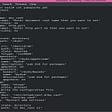# What happens when you type ls -l*.c in the shell?

• Ls : Prints to the screen a list of files and folders in the current folder the user is located
• -l : Display files and folders in long format. This option prints full folder contents including file permissions, bytes, ownership, file size, and total files and folders.
• *.c: The Ls command will only list those files that have a .c extension. The asterisk (*) is a wildcard, which I will detail on this soon.

# Let’s talk about inner workings.

`Alias lsm=’ls -l m*.c’`
`PATH=/usr/local/sbin:/usr/local/bin:/usr/sbin:/usr/bin:/sbin:/bin:/usr/games:/usr/local/games`
• /usr/local/sbin
• /usr/local/bin
• /usr/sbin
• /usr/bin
• /sbin
• /bin
• /usr/games
• /usr/local/games
`\$ printenvLC_PAPER=en_US.UTF-8XDG_SESSION_ID=5LC_ADDRESS=en_US.UTF-8LC_MONETARY=en_US.UTF-8TERM=xterm-256colorSHELL=/bin/bashSSH_CLIENT=10.0.2.2 53676 22LC_NUMERIC=en_US.UTF-8SSH_TTY=/dev/pts/0USER=vagrantLS_COLORS=rs=0:di=01;34:ln=01;36:mh=00:pi=40;33:so=01;35:do=01;35:bd=40;33;01:cd=40;33;01:or=40;31;01:su=37;41:sg=30;43:ca=30;41:tw=30;42:ow=34;42:st=37;44:ex=01;32:*.tar=01;31:*.tgz=01;31:*.arj=01;31:*.taz=01;31:*.lzh=01;31:*.lzma=01;31:*.tlz=01;31:*.txz=01;31:*.zip=01;31:*.z=01;31:*.Z=01;31:*.dz=01;31:*.gz=01;31:*.lz=01;31:*.xz=01;31:*.bz2=01;31:*.bz=01;31:*.tbz=01;31:*.tbz2=01;31:*.tz=01;31:*.deb=01;31:*.rpm=01;31:*.jar=01;31:*.war=01;31:*.ear=01;31:*.sar=01;31:*.rar=01;31:*.ace=01;31:*.zoo=01;31:*.cpio=01;31:*.7z=01;31:*.rz=01;31:*.jpg=01;35:*.jpeg=01;35:*.gif=01;35:*.bmp=01;35:*.pbm=01;35:*.pgm=01;35:*.ppm=01;35:*.tga=01;35:*.xbm=01;35:*.xpm=01;35:*.tif=01;35:*.tiff=01;35:*.png=01;35:*.svg=01;35:*.svgz=01;35:*.mng=01;35:*.pcx=01;35:*.mov=01;35:*.mpg=01;35:*.mpeg=01;35:*.m2v=01;35:*.mkv=01;35:*.webm=01;35:*.ogm=01;35:*.mp4=01;35:*.m4v=01;35:*.mp4v=01;35:*.vob=01;35:*.qt=01;35:*.nuv=01;35:*.wmv=01;35:*.asf=01;35:*.rm=01;35:*.rmvb=01;35:*.flc=01;35:*.avi=01;35:*.fli=01;35:*.flv=01;35:*.gl=01;35:*.dl=01;35:*.xcf=01;35:*.xwd=01;35:*.yuv=01;35:*.cgm=01;35:*.emf=01;35:*.axv=01;35:*.anx=01;35:*.ogv=01;35:*.ogx=01;35:*.aac=00;36:*.au=00;36:*.flac=00;36:*.mid=00;36:*.midi=00;36:*.mka=00;36:*.mp3=00;36:*.mpc=00;36:*.ogg=00;36:*.ra=00;36:*.wav=00;36:*.axa=00;36:*.oga=00;36:*.spx=00;36:*.xspf=00;36:LC_TELEPHONE=en_US.UTF-8MAIL=/var/mail/vagrantPATH=/usr/local/sbin:/usr/local/bin:/usr/sbin:/usr/bin:/sbin:/bin:/usr/games:/usr/local/gamesLC_IDENTIFICATION=en_US.UTF-8PWD=/home/vagrantLANG=en_US.UTF-8LC_MEASUREMENT=en_US.UTF-8PS1=\[\033[01;35m\](\T) \[\033[01;32m\]\u@\h\[\e\033[00m\]:\[\e\033[01;34m\]\W\[\e\033[00m\]\$(git_branch)\[\e\033[00m\]\n  ⟾  SHLVL=1HOME=/home/vagrantLOGNAME=vagrantSSH_CONNECTION=10.0.2.2 53676 10.0.2.15 22LESSOPEN=| /usr/bin/lesspipe %sXDG_RUNTIME_DIR=/run/user/1000LESSCLOSE=/usr/bin/lesspipe %s %sLC_TIME=en_US.UTF-8LC_NAME=en_US.UTF-8OLDPWD=/home/vagrant/simple_shell_=/usr/bin/printenv`

# So how does all this actually work?

`signal(SIGINT, sig_handler);`
`if (isatty(STDIN_FILENO))`

# execve()

`if (execve("./sub", argv, envp) == -1){    perror("Could not execve");    return 1;}`

# Exit

--

--

--

## More from Sergio Vera

Love podcasts or audiobooks? Learn on the go with our new app.

## Hidden Secrets of Dockerfile## Understanding Async/Await in C### The real benefit of 100% code coverage## Database integration tests in local environment## Asp.Net MVC 5 Interview Questions and Answers | Part-2## C Static Library Simplified## Observe + Quantify